CBSE Class 10 Sample Paper for 2021 Boards - Maths Standard

Class 10
Solutions of Sample Papers for Class 10 Boards

## The two palm trees are of equal heights and are standing opposite each other on either side of the river, which is 80 m wide. From a point O between them on the river the angles of elevation of the top of the trees are 60° and 30°, respectively. Find the height of the trees and the distances of the point O from the trees.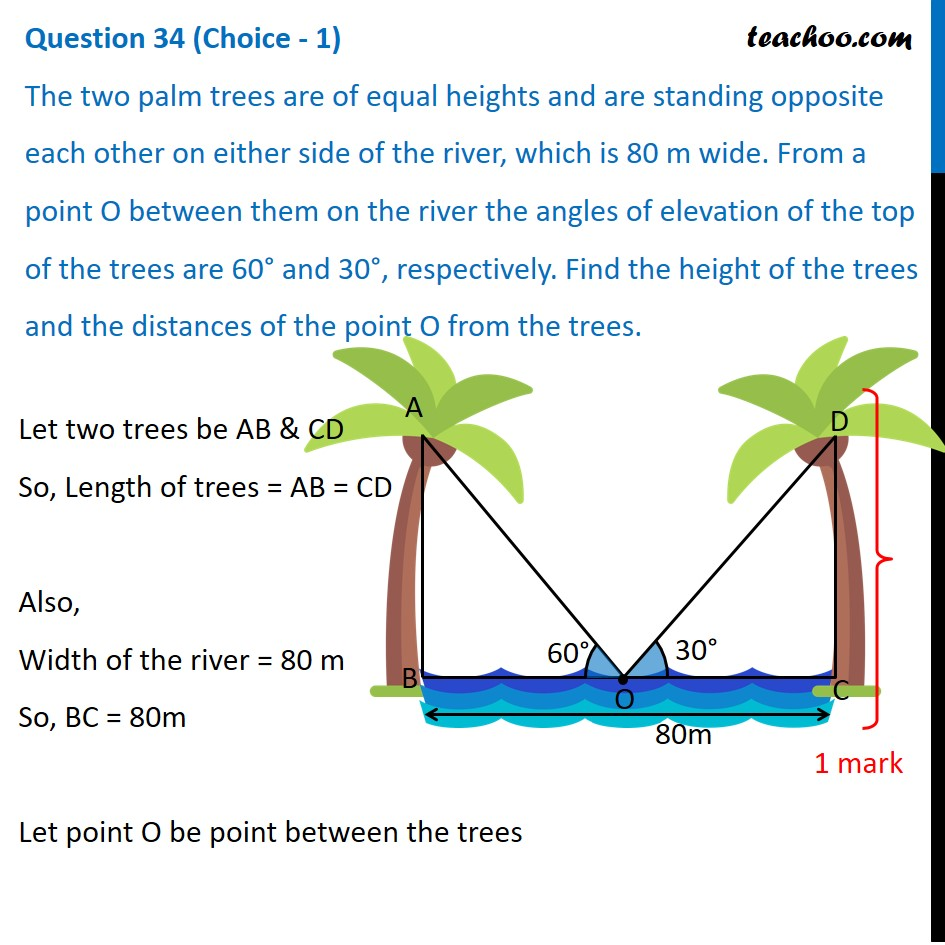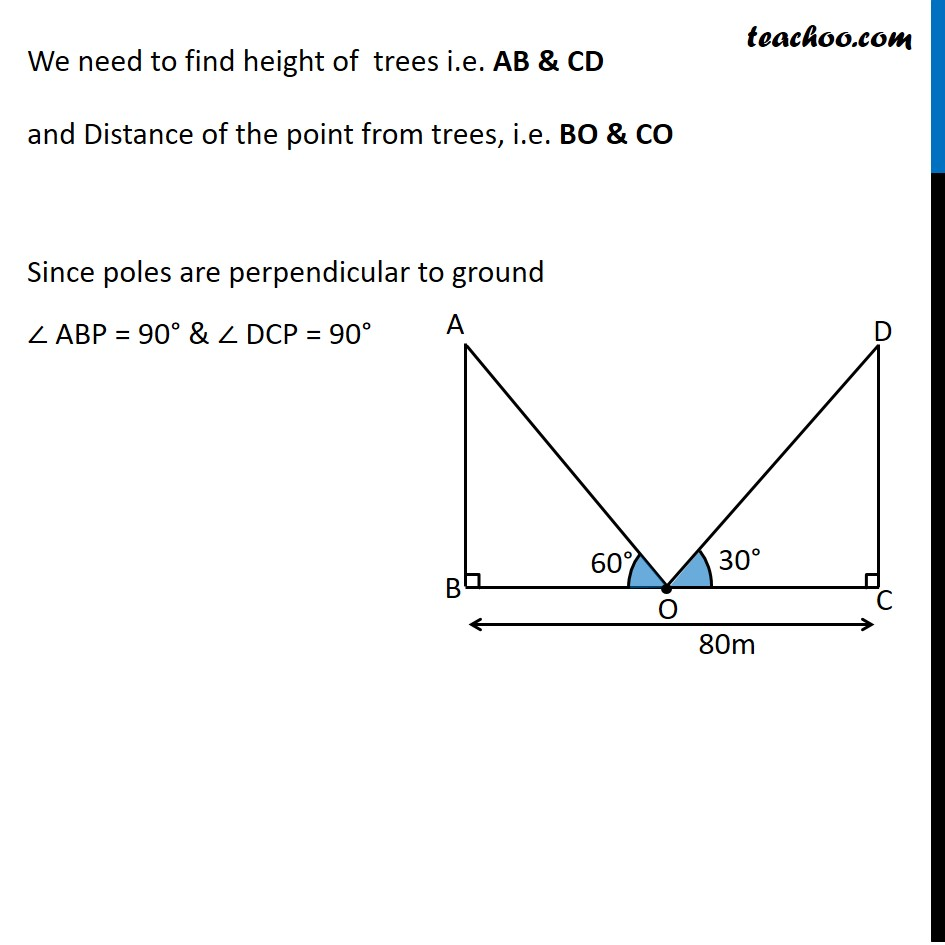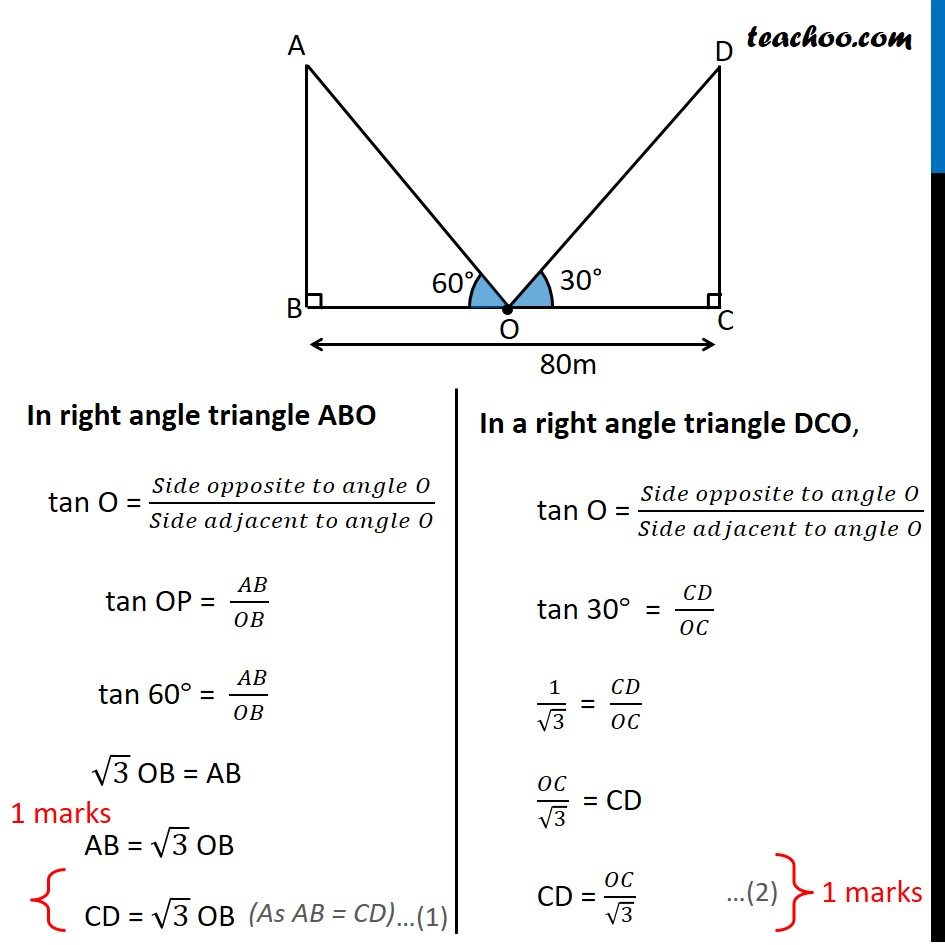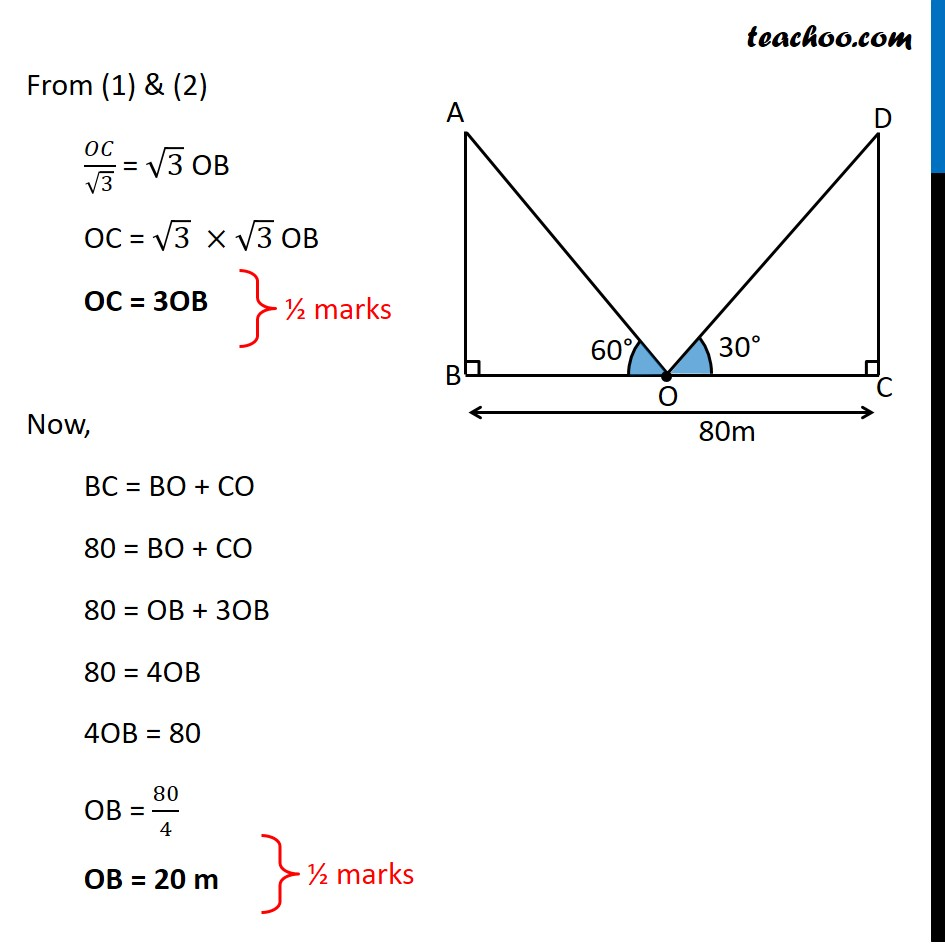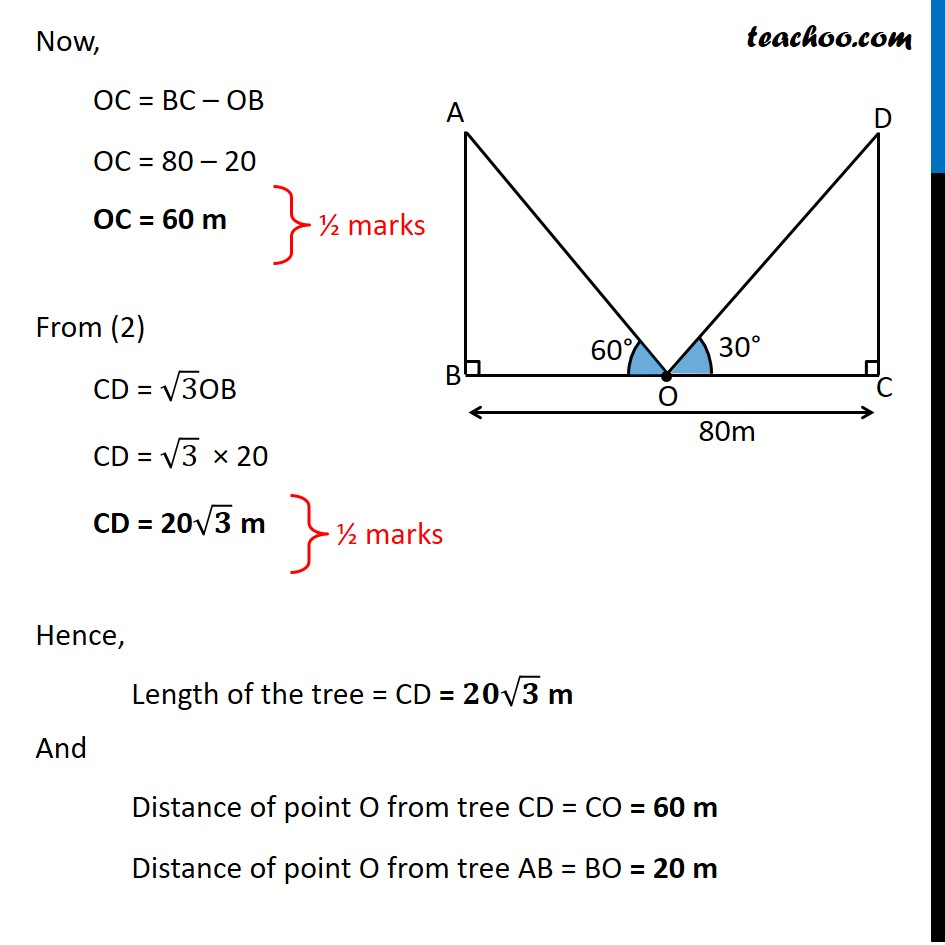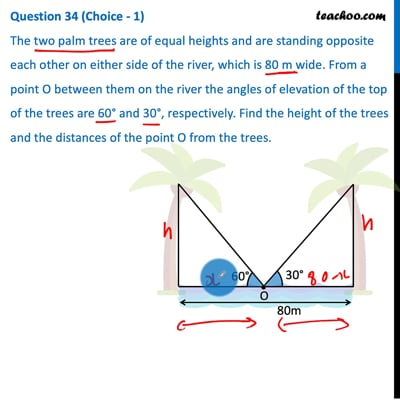This video is only available for Teachoo black users

Note : This is similar to Ex 9.1, 10,  Chapter 9 Class 10 Applications of Trigonometry (NCERT Book)

Introducing your new favourite teacher - Teachoo Black, at only ₹83 per month

### Transcript

Question 34 (Choice - 1) The two palm trees are of equal heights and are standing opposite each other on either side of the river, which is 80 m wide. From a point O between them on the river the angles of elevation of the top of the trees are 60° and 30°, respectively. Find the height of the trees and the distances of the point O from the trees.Let two trees be AB & CD So, Length of trees = AB = CD Also, Width of the river = 80 m So, BC = 80m Let point O be point between the trees We need to find height of trees i.e. AB & CD and Distance of the point from trees, i.e. BO & CO Since poles are perpendicular to ground ∠ ABP = 90° & ∠ DCP = 90° In right angle triangle ABO tan O = (𝑆𝑖𝑑𝑒 𝑜𝑝𝑝𝑜𝑠𝑖𝑡𝑒 𝑡𝑜 𝑎𝑛𝑔𝑙𝑒" " 𝑂)/(𝑆𝑖𝑑𝑒 𝑎𝑑𝑗𝑎𝑐𝑒𝑛𝑡 𝑡𝑜 𝑎𝑛𝑔𝑙𝑒" " 𝑂) tan OP = (" " 𝐴𝐵)/𝑂𝐵 tan 60° = (" " 𝐴𝐵)/𝑂𝐵 √3 OB = AB AB = √3 OB CD = √3 OB In a right angle triangle DCO, tan O = (𝑆𝑖𝑑𝑒 𝑜𝑝𝑝𝑜𝑠𝑖𝑡𝑒 𝑡𝑜 𝑎𝑛𝑔𝑙𝑒" " 𝑂)/(𝑆𝑖𝑑𝑒 𝑎𝑑𝑗𝑎𝑐𝑒𝑛𝑡 𝑡𝑜 𝑎𝑛𝑔𝑙𝑒" " 𝑂) tan 30° = (" " 𝐶𝐷)/𝑂𝐶 (" " 1)/√3 = 𝐶𝐷/𝑂𝐶 𝑂𝐶/√3 = CD CD = 𝑂𝐶/√3 From (1) & (2) 𝑂𝐶/√3 = √3 OB OC = √3 ×√3 OB OC = 3OB Now, BC = BO + CO 80 = BO + CO 80 = OB + 3OB 80 = 4OB 4OB = 80 OB = 80/4 OB = 20 m Now, OC = BC – OB OC = 80 – 20 OC = 60 m From (2) CD = √3OB CD = √3 × 20 CD = 20√𝟑 m Hence, Length of the tree = CD = 𝟐𝟎√𝟑 m And Distance of point O from tree CD = CO = 60 m Distance of point O from tree AB = BO = 20 m